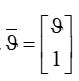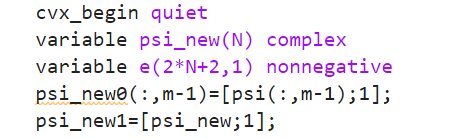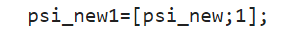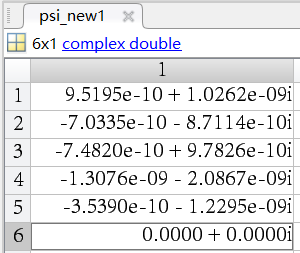# As shown in the figure, the psi _ new variable is defined in cvx, and the psi _ new1 variable in Figure 2 represents the variable on the left side of the equation in Figure 1. The results show that the N + 1 variable in psi _ new1 is not 1. Why is this?As shown in the figure, the psi _ new variable is defined in cvx, and the psi _ new1 variable in Figure 2 represents the variable on the left side of the equation in Figure 1. The results show that the N + 1 variable in psi _ new1 is not 1. Why is this ?

Please properly formulate your question. Do not refer to figures which have not been provided.

Do not use `quiet`until you are sure everything is working correctly.

Declare `psi_new0` as an expression of the appropriate dimensions before assigning values to it. See The Basics — CVX Users' Guide .

`psi_new1` is an expression, because it is set with `=`. After CVX completes, expressions are not necessarily populated with their “optimal” values, even though the “optimal” values are “used” during the optimization. Therefore, if the optimal expression values are needed, they must be recomputed after cvx_end, starting with CVX variable values.I want to represent psi _ ba in the equation so that the N + 1 element is fixed to 1

`g_bar = [g;1];` should accomplish that.

I tried like this:But the result is：why？

It is very hard to help without having a reproducible example, that is a piece of code we can copy-paste, run, and where you indicate what you think is wrong. We simply don’t know what you are doing and in what order and where the problems appear.